## Measuring battery voltage with Nordic nRF51x devices

When running embedded applications on devices powered by battery, it is very common to read the input voltage and use this information to determine which task will be executed next. In addition, low-power microcontrollers usually have a low pin count and these lines have to be used effectively. A few will be used to "talk" to peripherals to read from sensors and write to actuators, meanwhile others are simple required to get the microcontroller up and running (power supply, clk / crystal, reset-logic, etc).

From the Nordic nRF51x Reference Manual, we found out that the SoC contains a VBG (internal 1.2 V band gap reference) that can be wired to the ADC as voltage reference, in addition to the Vcc (power-supply) connected to the ADC as analog input.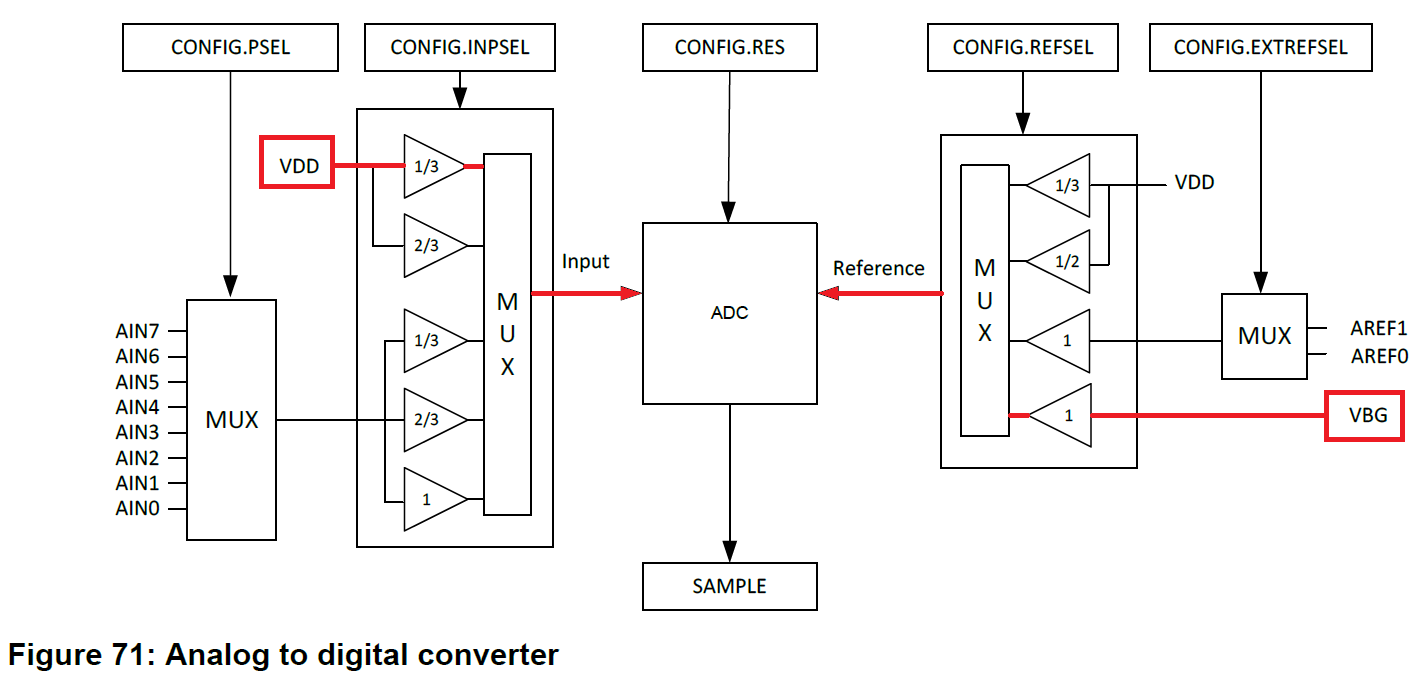This is a very interesting feature, as it let us dedicate an analog input for a different purpose or simple reduce the BoM & costs. In addition, we could potentially detect whether the device is being powered over USB or a coin-cell battery, depending on the value provided by the ADC. For example, from the nRF51-DK board schematic, we can see the power supply circuit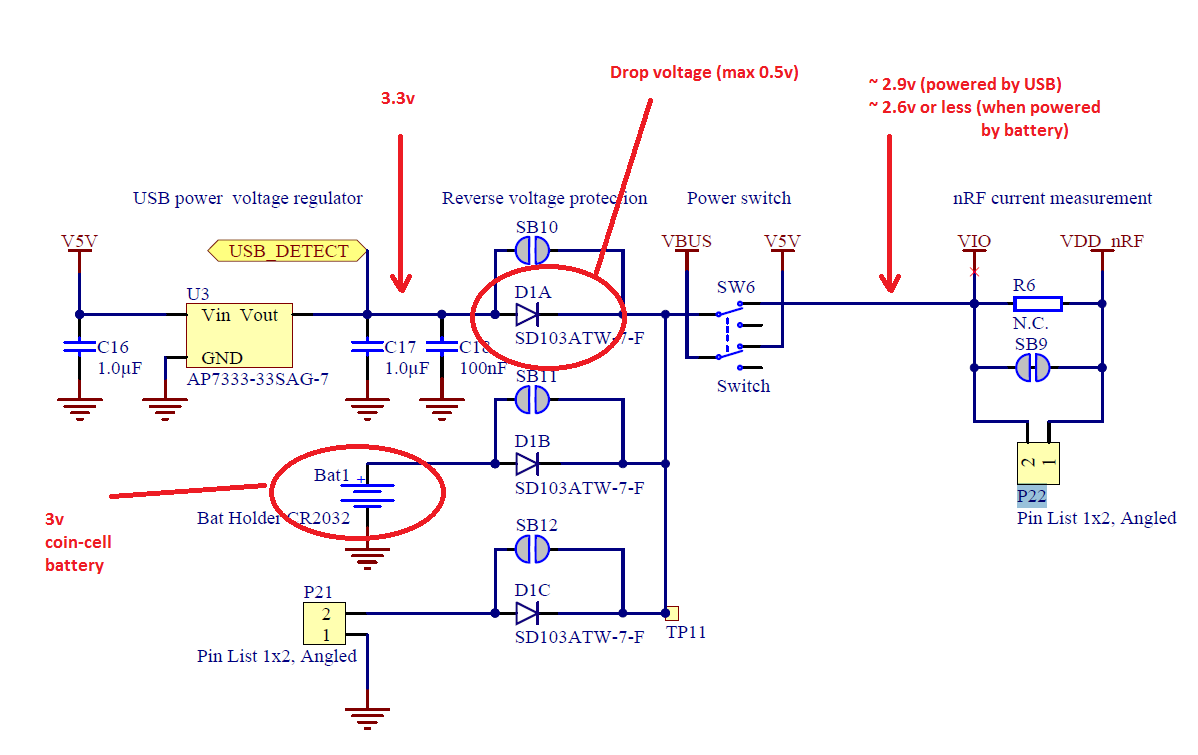A simple program has been created to proof this concept and show a real example:

```/*
* This is a simple program that let us use the ADC to read the input voltage.
*/

#include "mbed.h"

DigitalOut led_status(LED3);
Serial device(p9, p11);  // tx, rx

{
}

{
}

int main()
{
float value;

device.baud(115200);

while(1) {

led_status = 0;
wait(0.2);
led_status = 1;
wait(0.8);

value = (value * 3.6) / 1024.0;
device.printf("Input Voltage: %f\n\r",value);
}
}
```

We used an adjustable power supply to perform the tests: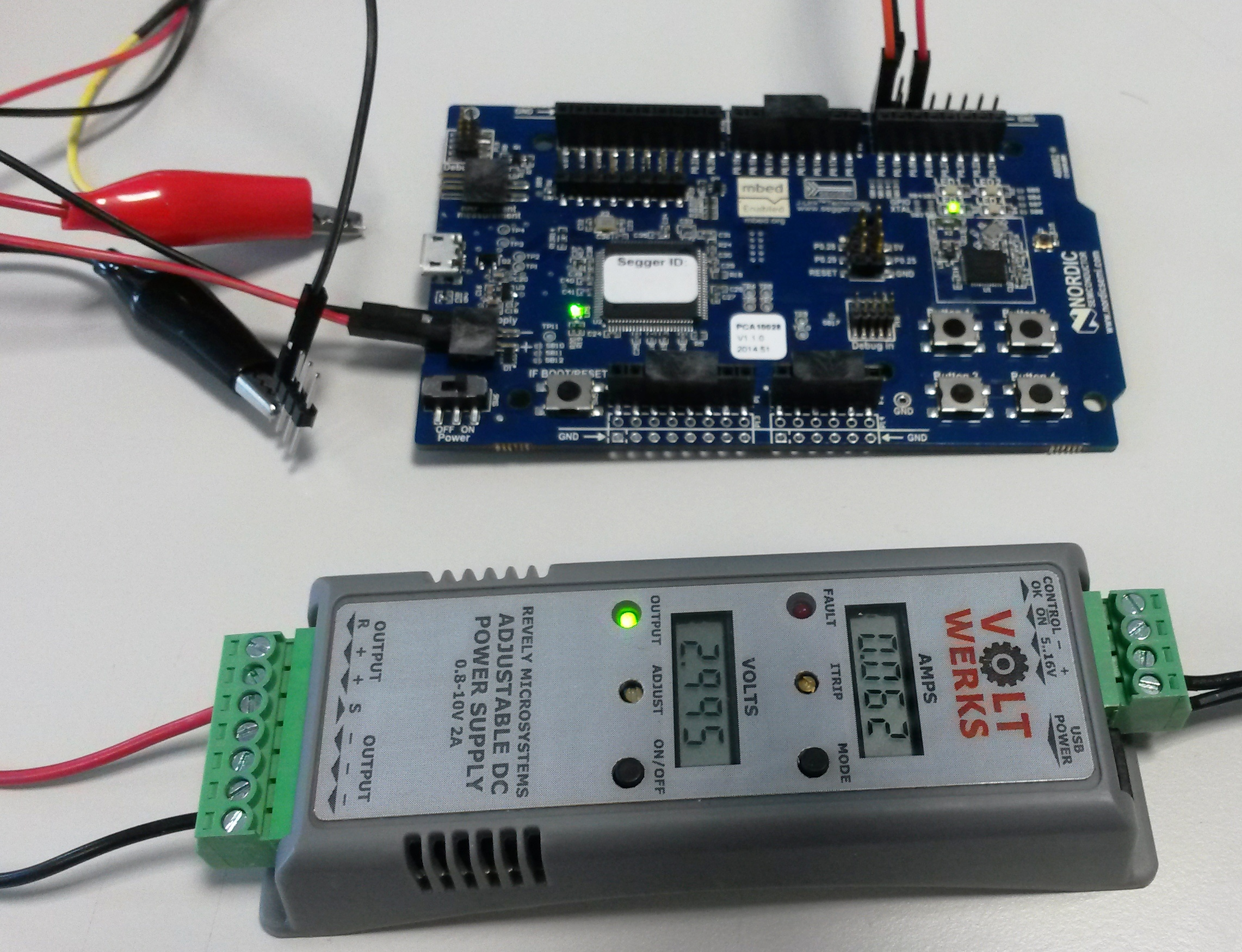This is the output from the serial console: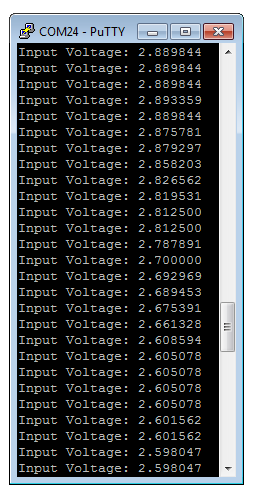### 4 comments on Measuring battery voltage with Nordic nRF51x devices:

Hi,

I tried your code and it looks really good. I was wondering though, when I changed the ADC resolution from 10 to 8 and 9 bits, it gave different voltage readings. For 10 bits it gave 2.6V, 8 bits gave 0.8V, and 9 bits gave 1.45V. Any reason why?

why is it multiplied by 3.6 volts and not 3V which is the max of coin cell. I still get a wrong value. I am using your program and I get 2.68 volts while battery is is a 2.90 volts. if I put 3 volts instead of 3.6 volts my reading even falls further down.

####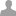Sriram Boppana wrote:

Hi,

I tried your code and it looks really good. I was wondering though, when I changed the ADC resolution from 10 to 8 and 9 bits, it gave different voltage readings. For 10 bits it gave 2.6V, 8 bits gave 0.8V, and 9 bits gave 1.45V. Any reason why?

It simple, as resolution changes, max possible value change as well.

For 10 bit max possible value is 1024, and for 8 bit max possible value is 255. So for 8bit you will need to use this formula. value = (value * 3.6) / 255.0;

Hello

Great lib, but it is possible to get this to work on mbed-os 5.10?

I get Error: Identifier "NRF_ADC" is undefined in "main.cpp",

Thanks